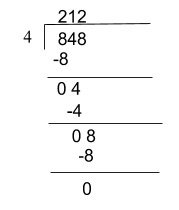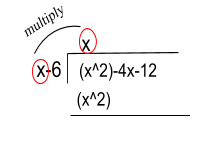# Algebraic Long Division

Contents

#### Summary

• Polynomial long division is normal long division but with polynomials instead of just numbers.
• If a polynomial p(x) is to be divided by (x – a) and p(a) = 0 then we can say that (x – a) is a factor of the polynomial
• Revise the steps below to be able to carry out algebraic long division.

Algebraic long division is really useful to divide polynomials by polynomials and work out the quotient and the remainder.

Polynomial long division is nat our normal quotients of numbers do. To start with, let’s review the process of our usual long division. We will then extend to polynomials.

Q. Solve$848\quad \div \quad 4\$

Solution:The remainder here is 0, and the quotient is 212.

Just like we did the above long division, we use the same concept for dividing polynomials. In algebraic long division the terms consist of xnand thus the quotient and the remainder are also in terms of x.

Let’s go through an example to understand what steps are carried out while performing algebraic long division.

#### Example #1

Q. Divide$-4x\quad +\quad { x }^{ 2 }-\quad 12\quad by\quad (x-6)$

Solution:

Step 1: Make sure that the terms in the polynomial are in descending order i.e$-4x\quad +\quad { x }^{ 2 }-\quad 12$

Rearrange${ x }^{ 2 }\quad -4x\quad -\quad 12$

Step 2: Perform the division, start off with dividing the first term of the polynomial by the first term of the divisor i.eStep 3: Since we have the first term of the quotient now, we can multiple this term with the second term of the divisor i.eStep 4: This step is similar to what we do at this stage when dividing numbers only. We subtract the first two terms in the dividend from the term at the bottom i.eStep 5: Bring the next term from the dividend to the remainder after subtraction.Step 6: Repeat the above steps for this remainder term to find the next term of the quotient and further continue the process until a remainder is obtained. This can be zero or of lower index than the divisor i.e

Note: If a polynomial p(x) is to be divided by (x – a) and p(a) = 0 then we can say that (x – a) is a factor of the polynomial and “a” is a root of p(x). The remainder on division is also 0 if and only if (x – a) is a factor of p(x).

Therefore, in the above example we can say that as p(6)=0 hence, (x-6) is a factor of the polynomial${ x }^{ 2 }\quad -4x\quad -\quad 12$

#### Example #2

Suppose we are given a polynomial$p(x)\quad =\quad 2{ x }^{ 3 }\quad +\quad 8{ x }^{ 2 }\quad -\quad 3x\quad -\quad 12$

To divide this we require a divisor. We can check that by putting x = -4, we get p(-4) = 0. Hence, (x + 4) is a factor of the polynomial so we can divide the polynomial by it.

The division is carried out in the following way:Quotient =$2{ x }^{ 2 }\quad -\quad 3$ and as the remainder is 0, it confirms that (x + 4) is a factor of the polynomial.

##### Reference
1. http://mathematics.laerd.com/maths/algebraic-division-intro.php
2. https://www.tamdistrict.org/cms/lib/CA01000875/Centricity/Domain/539/Polynomial%20Long%20Division%20w-remainder%20Zero%20Notes.pdf
3. https://www.math.ucdavis.edu/~tlazarus/files/poly_long_div.pdf